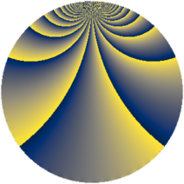# Properties

 Label 1815.2.kLevel $1815$ Weight $2$ Character orbit 1815.k Rep. character $\chi_{1815}(122,\cdot)$ Character field $\Q(\zeta_{4})$ Dimension $400$ Sturm bound $528$

# Related objects

## Defining parameters

 Level: $$N$$ $$=$$ $$1815 = 3 \cdot 5 \cdot 11^{2}$$ Weight: $$k$$ $$=$$ $$2$$ Character orbit: $$[\chi]$$ $$=$$ 1815.k (of order $$4$$ and degree $$2$$) Character conductor: $$\operatorname{cond}(\chi)$$ $$=$$ $$15$$ Character field: $$\Q(i)$$ Sturm bound: $$528$$

## Dimensions

The following table gives the dimensions of various subspaces of $$M_{2}(1815, [\chi])$$.

Total New Old
Modular forms 576 472 104
Cusp forms 480 400 80
Eisenstein series 96 72 24

## Trace form

 $$400q + 8q^{6} + O(q^{10})$$ $$400q + 8q^{6} - 20q^{12} + 16q^{13} - 12q^{15} - 288q^{16} + 12q^{18} + 16q^{21} - 12q^{27} - 32q^{28} - 16q^{30} + 32q^{31} - 24q^{36} + 48q^{37} - 8q^{40} + 4q^{42} - 28q^{45} - 16q^{48} - 16q^{51} - 88q^{52} - 40q^{57} + 16q^{58} + 168q^{60} - 4q^{63} - 40q^{67} + 56q^{70} - 48q^{72} + 52q^{75} - 96q^{76} + 20q^{78} + 72q^{81} + 8q^{82} + 8q^{85} - 16q^{87} + 16q^{90} + 48q^{91} - 76q^{93} - 128q^{96} - 88q^{97} + O(q^{100})$$

## Decomposition of $$S_{2}^{\mathrm{new}}(1815, [\chi])$$ into newform subspaces

The newforms in this space have not yet been added to the LMFDB.

## Decomposition of $$S_{2}^{\mathrm{old}}(1815, [\chi])$$ into lower level spaces

$$S_{2}^{\mathrm{old}}(1815, [\chi]) \cong$$ $$S_{2}^{\mathrm{new}}(165, [\chi])$$$$^{\oplus 2}$$